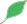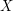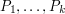## If you don't know how to make a decision, then don't make itThis article is a stub.This means that it cannot be considered to contain or lead to any mathematically interesting information.

### Quick description

Very often a proof requires one to choose some object that will make the rest of the proof work. And very often it is far from obvious how to make the choice. Often a good way of getting round the problem is to take an arbitrary object of the given type, give it a name, and continue with the proof as if you had chosen. Along the way, you will find that you need to assume certain propertiesof. Then your original problem is reduced to the question "Does there exist an object of the given type with properties?" Often, this is a much more straightforward question than the main problem you were trying to solve.

A very simple example would be doing an analysis argument where a certain parameter has to be small enough. But it would be nice to have some much more structural examples. An example already appears in the article How to solve cubic and quartic equations in the course of the discussion of the general solution of a cubic.